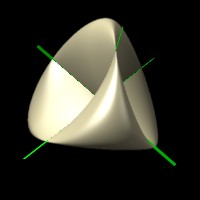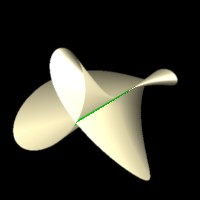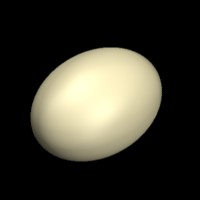Movie of the week - Week 3The images above comprise the frames of the Week 3 algebraic geometry movie of the week. Each is an affine part of the real locus of the projection of the Veronese surface from projective 5 space to projective 3 space. The Veronese surface is the image of the projective plane using the 6 degree 2 monomials. The image of projection from a line in P^5 gives a projective variety in P^3. The real locus of the intersection of this projection with an affine 3 space is drawn in the pictures above, which are all projectively inequivalent over the complex field. Any complex projection is projectively equivalent to one of the 6 above or lies in a plane in 3 space. The green lines are contained in the surface (any line hitting a hypersurface in P^3 more times than its degree is wholly contained in the surface), and are the singular locus of the variety, that is points where the gradient of the defining polynomial vanishes.

These are all related to examples of Steiner surfaces, which are the images of the real projective plane in P^3 given by 4 independent homogeneous polynomials of degree 2 in 3 variables. The real locus of the projection contains the projection of the real locus, but in some cases is strictly bigger. In these pictures any green lines not in the main surface are in fact not in the projection of the real projective plane, but are in the real locus of the projection of the Veronese surface. The left most is Steiner's Roman surface, while the second from right is the Caley ruled cubic surface. Visit A. Coffman's Steiner Surface page for a nice source of information on these objects, as well as a discussion of the classification over the real field.

The general projection will yield a variety projectively equivalent to the Roman surface. When projecting from a line L in P^5 which misses the Veronese surface to a dimension 3 linear space disjoint from the line we compute where the plane determined by L and a point on the Veronese hits the dimension 3 space. This map is one to one except when a plane hits the Veronese in more than one point, in which case the secant line joining these points must hit L at a point where L hits the set of all secant lines. This secant variety is a hypersurface in P^5 given by a degree 3 homogeneous polynomial (see Harris, page 145), so there are 3 points on L that also lie on a secant to the Veronese surface. For each of these three points there is a P^1 of lines through them that hit the Veronese 2 times, and they all live in a plane hitting L only at 1 point. (see Harris picture on page 144). For planes determined by L and these lines we get 2 points on the Veronese mapping to the same point on the projection, and these image points lie in a linear P^1 in three space. Thus the general projection to P^3 will have 3 double lines, which by similar logic will intersect in a point. This explains geometrically the 3 green lines meeting at the center of the picture of Steiner's Roman Surface.

We list the 6 different lines that we project from to get the 6 examples above. See the Macaulay worksheet 3 to see how to compute the equations of the projections. Take the Veronese as the set of points [z_0,...,z_5]=[u_0^2,u_1^2,u_2^2,u_0u_1,u_0u_2,u_1u_2]. The lines are

z_0+z_1+z_2=z_3=z_4=z_5=0

z_3=z_4=z_2-z-1=z-0-z_1+z_2=0

z_0+2z_1+z_2=2z_1+z_2=z_2+z_4=z_3+z_5=0

z_3=z_4=z_5=z_1-z_2=0

z_3=z_4-z_1=z_5=z_2=0

z_0+z_1+z_2=z_3=z_4=z_0-z_1-z_2=0

The last is described topologically by identifying the equator to a point in the upper hemisphere model of the projective line, which yields a topological sphere.